# Python | Pandas DataFrame.ix[ ]

Python is a great language for doing data analysis, primarily because of the fantastic ecosystem of data-centric Python packages. Pandas is one of those packages and makes importing and analyzing data much easier.

Pandas `DataFrame.ix[ ]` is both Label and Integer based slicing technique. Besides pure label based and integer based, Pandas provides a hybrid method for selections and subsetting the object using the `ix[]` operator. `ix[]` is the most general indexer and will support any of the inputs in `loc[]` and `iloc[]`.

Syntax: DataFrame.ix[ ]

Parameters:
Index Position: Index position of rows in integer or list of integer.
Index label: String or list of string of index label of rows

Returns: Data frame or Series depending on parameters

Code #1:

 `# importing pandas package  ` `import` `pandas as geek ` `   `  `# making data frame from csv file ` `data ``=` `geek.read_csv(``"https://media.geeksforgeeks.org/wp-content/uploads/nba.csv"``)   ` `   `  `# Integer slicing ` `print``(``"Slicing only rows(till index 4):"``) ` `x1 ``=` `data.ix[:``4``, ] ` `print``(x1, ``"\n"``) ` `  `  `print``(``"Slicing rows and columns(rows=4, col 1-4, excluding 4):"``) ` `x2 ``=` `data.ix[:``4``, ``1``:``4``] ` `print``(x2) `

Output :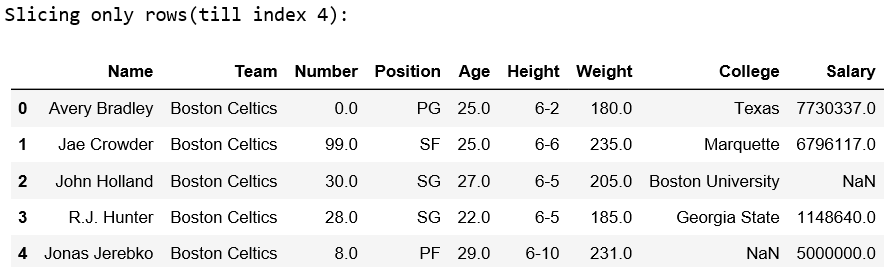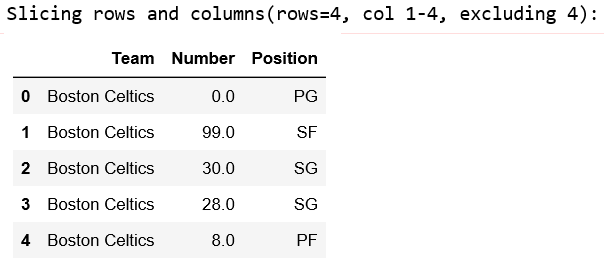Code #2:

 `# importing pandas package  ` `import` `pandas as geek ` `   `  `# making data frame from csv file ` `data ``=` `geek.read_csv(``"nba.csv"``)   ` `   `  `# Index slicing on Height column ` `print``(``"After index slicing:"``) ` `x1 ``=` `data.ix[``10``:``20``, ``'Height'``] ` `print``(x1, ``"\n"``) ` ` `  `# Index slicing on Salary column ` `x2 ``=` `data.ix[``10``:``20``, ``'Salary'``] ` `print``(x2) `

Output: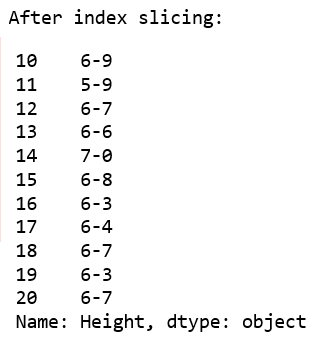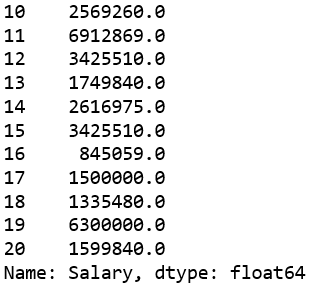Code #3:

 `# importing pandas and numpy ` `import` `pandas as pd ` `import` `numpy as np ` `  `  `df ``=` `pd.DataFrame(np.random.randn(``10``, ``4``), ` `          ``columns ``=` `[``'A'``, ``'B'``, ``'C'``, ``'D'``]) ` ` `  `print``(``"Original DataFrame: \n"` `, df) ` `  `  `# Integer slicing ` `print``(``"\n Slicing only rows:"``) ` `print``(``"--------------------------"``) ` `x1 ``=` `df.ix[:``4``, ] ` `print``(x1) ` `  `  `print``(``"\n Slicing rows and columns:"``) ` `print``(``"----------------------------"``) ` `x2 ``=` `df.ix[:``4``, ``1``:``3``] ` `print``(x2) `

Output :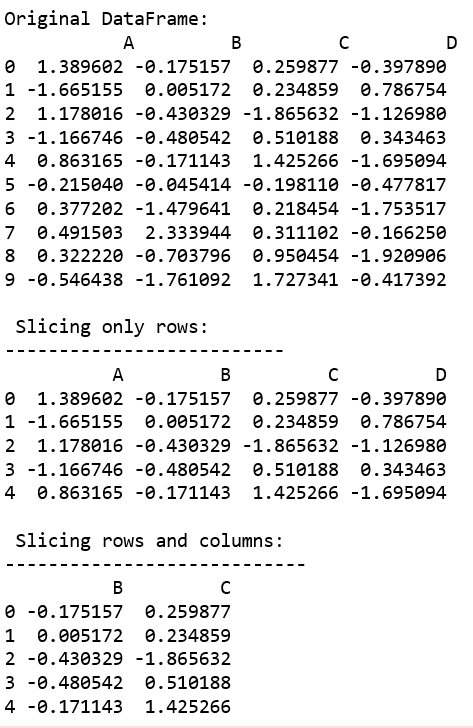Code #4:

 `# importing pandas and numpy ` `import` `pandas as pd ` `import` `numpy as np ` `  `  `df ``=` `pd.DataFrame(np.random.randn(``10``, ``4``), ` `          ``columns ``=` `[``'A'``, ``'B'``, ``'C'``, ``'D'``]) ` ` `  `print``(``"Original DataFrame: \n"` `, df) ` `  `  `# Integer slicing (printing all the rows of column 'A') ` `print``(``"\n After index slicing (On 'A'):"``) ` `print``(``"--------------------------"``) ` `x ``=` `df.ix[:, ``'A'``] ` ` `  `print``(x) `

Output :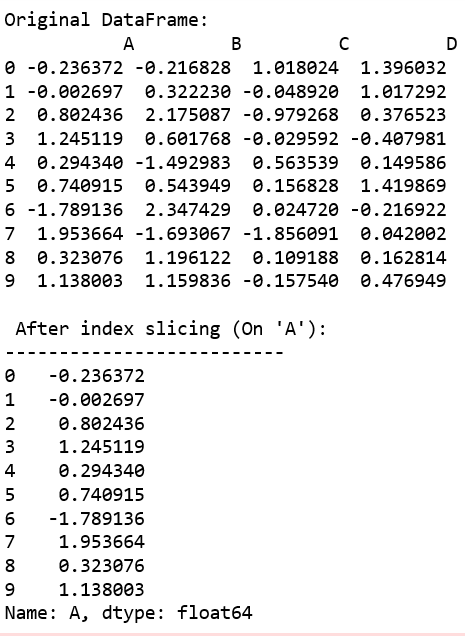Whether you're preparing for your first job interview or aiming to upskill in this ever-evolving tech landscape, GeeksforGeeks Courses are your key to success. We provide top-quality content at affordable prices, all geared towards accelerating your growth in a time-bound manner. Join the millions we've already empowered, and we're here to do the same for you. Don't miss out - check it out now!

Previous
Next